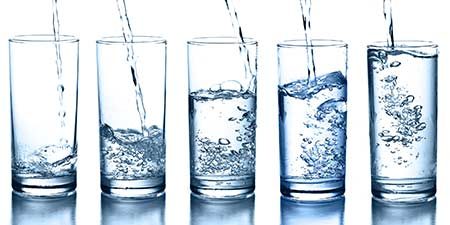# Cylinder Calculation - Calculation of Volume, Lateral Surface Area, Total Surface Area, Circumference of the Base and Diameter

Cylinder ﹣ Calculator

How do you calculate the volume of a cylinder? What is the lateral (curved) surface area (LSA) of a cylinder? What is the total surface area (TSA) of a cylinder? Here you will find everything you need to know about cylinders: definitions, formulas and calculations for the volume, the lateral surface area, the total surface area, the cylinder height, as well as the radius, diameter and circumference of the base of a cylinder. With the help of the cylinder calculator, you can calculate all of these cylinder properties, from the height to the volume of the cylinder to the cylinder base area. Each of the cylinder properties is then clearly derived using calculation examples with the help of the appropriate cylinder formulas.

## Input Help for the Cylinder Calculator for Calculating Volume, Base Area, Height, Surface Areas and more

The cylinder calculation calculator contains several input fields, which are explained in more detail below:

### Size unitPlease select the appropriate unit of measurement to display the corresponding input fields and results. You can choose from mm, in, dm, m, and km.

### Select the first cylinder property: Radius, diameter, circumference or base areaPlease select one of the four properties (radius, diameter, circumference of the base, or area) and enter the value for it. The calculation of cylinders includes the calculation of the two base surfaces. In the example of a beverage can, the base and the lid form these base surfaces. For the circle calculation required here to calculate the circular cylinder base surfaces, it is sufficient if one of the four properties is known.

### Select the second cylinder property: Height, volume or surface areaPlease select one of the three properties height, volume or surface area and enter the value. After calculating the circular base area using radius, diameter, circumference of the base, or surface area, it is necessary to enter another property in order to calculate the cylinders surface area and subsequently all geometric properties of the cylinder. For a complete cylinder calculation, it is sufficient if one of the three properties height, volume or surface area is known.

### SketchThe sketch shows you the combination of the two selected cylinder properties. This gives you an idea of how the radius, diameter, circumference of the base, base area, height, volume and surface area of the cylinder are defined. The display changes as soon as you select another cylinder property.

## Definition of a CylinderA cylinder (circular cylinder) is a geometric body. It is formed by two parallel circular bases of equal size connected by a mantle (curved surface). Typical examples of cylindrical shapes are a beverage can, a grain silo or a drinking glass.

Important properties of a cylinder are the radius, the diameter, the circumference of the base, the base area, the cylinder height, the volume of the cylinder, the lateral surface area (LSA) and the total surface area (TSA) of the cylinder. The calculation of these cylinder properties and their conversion using cylinder formulas can be conveniently carried out with the cylinder calculator, as will be discussed further below.

## The Radius of a Cylinder

The radius of the cylinder's circular base surface is the distance between the centre and the outer edge of this base surface. The cylinder radius thus describes the distance between the cylinder's centre axis and the surrounding lateral surface.The cylinder radius r, also known as the radius, is the distance between the cylinder's axis (i.e., the centre of the cylinder's base surface) and the curved surface.

## Cylinder Diameter - Definition and Formula

### Definition of Cylinder DiameterThe cylinder diameter d or ⌀ is the length of the connecting distance between two points on the circular line through the centre M of the cylinder base.

The diameter thus corresponds to the "thickness" of a cylinder.

### Formula for Cylinder Diameter

The diameter d or ⌀ of the cylinder corresponds to its twofold radius r.

d = 2 × r

## Cylinder Circumference - Definition and Formula

### Definition of Circumference of the Cylinder BaseThe circumference of the base C of a cylinder is the distance around the circular edge of the base of the cylinder.

### Formula for Circumference of the Cylinder Base

The circumference of the base C of a cylinder is calculated by multiplying the cylinder diameter d by the number Pi (π = 3.1415...).

C = 2 × r × π = d × π

## Cylinder Base Area - Definition and Formula

### Definition of Cylinder Base Area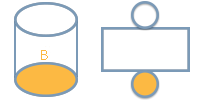The base area B is the area enclosed by the cylinder surface. The base area of the cylinder is actually the area of a circle, since the base of the cylinder is a circle.

### Formula for Cylinder Base Area

The base area B is calculated by multiplying the square of the cylinder radius by Pi (π = 3.1415...).

B = r² × π

## The Height of a Cylinder

The height h of a cylinder corresponds to the distance between its two parallel base surfaces. Using the example of the beverage can, the cylinder height corresponds to the height of the can.

### Definition of Cylinder Height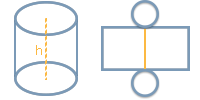The height h of a cylinder corresponds to the distance between the two parallel cylinder bases.

## Cylinder Volume - Definition and Formula

### Definition of Cylinder Volume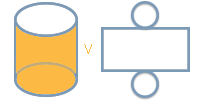The cylinder volume V describes the spatial expansion of a cylinder.

### Formula for Cylinder Volume

The volume V of a cylinder is obtained by multiplying the base area B by the cylinder height h.

V = B × h

## Cylinder Lateral Surface Area - Definition and Formula

### Definition of Cylinder Lateral Surface AreaThe development of the cylinder surface area LSA (Curved Surface Area or Lateral Surface Area) is a rectangle whose length and width correspond to the height h of the cylinder and the circumference of the base C of the cylinder.

### Formula for Cylinder Lateral Surface Area

The lateral surface area LSA corresponds to the product of the cylinder height h and the cylinder circumference of the base C and is therefore calculated using the following formula.

LSA = h × C

## Cylinder Total Surface Area - Definition and Formula

### Definition of Cylinder Total Surface AreaThe total surface area TSA of a cylinder is made up of twice the base area B and the lateral surface area LSA of the cylinder.

### Formula for Cylinder Total Surface Area

The total surface area TSA of a cylinder is equal to twice the base area B plus the lateral surface area LSA.

TSA = 2 × B + LSA

## Cylinder Calculation Examples

To calculate a cylinder, two known values are required: First, a value must be given for the calculation of the cylinder base area, i.e., either the radius, the diameter, the circumference of the base, or the base area itself. Then one of the three values for the cylinder height, volume, or lateral surface area must be known in order to calculate the other two together with the already calculated base area and finally the cylinder total surface area.

Therefore, in the following sections 1.1 to 1.4, we will first show you 12 examples of calculations involving the cylinder base area. These are the first three examples of how the cylinder radius can be calculated using the diameter, the circumference of the base, and the base area. This is followed by three examples of how to determine the cylinder diameter, three examples of how to determine the circumference of the cylinder base, and finally three examples of how to determine the base area using the other three cylinder properties in each case.

Subsequently, under sections 2.1 to 2.4, further examples are provided for calculating the entire cylinder using the now-known base area and the second known value, i.e., height, volume, or surface area.

The following three examples show the calculation of the cylinder radius using the cylinder properties (diameter, circumference of the base, and base area).

### How do you calculate the radius of a cylinder from its diameter?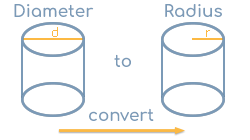Given a cylinder with a diameter d of 5 in. We are looking for the radius r of the cylinder.

#### Calculation

The cylinder radius r corresponds to half the diameter d, i.e., r = d / 2.

If the 5 in chosen in the example are used for the diameter d, the radius of the cylinder is r =  5 in / 2 = 2.5 in.

### How do you calculate the radius of a cylinder from the circumference of the base?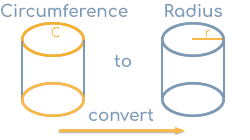Given a cylinder with a circumference of the base C of 15 in. We are looking for the radius r of the cylinder.

#### Calculation

The formula for the cylinder circumference of the base C is C = 2 × r × π. If we convert this formula to r, the radius r is equal to half the division of the circumference of the base divided by pi (π = 3.1415...), i.e., r = C / π / 2.

If we substitute the 15 in chosen in the example for the circumference of the base C, the radius of the cylinder is r = 15 in / π / 2 ≈ 2.39 in.

### How do you calculate the radius of a cylinder from the base area?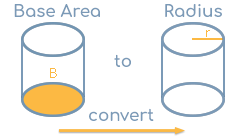Let a cylinder with a base area B of 20 in² be given. We are looking for the radius r of the cylinder.

#### Calculation

The general formula for the base area B is B = r² × π. If we convert this formula to r, the radius r corresponds to the square root of the result of the base area B divided by Pi (π = 3.1415...), i.e., r = B / π.

If the 20 in² chosen in the example are used for the base area B, the radius of the cylinder is r = 20 in² / π ≈ 2.52 in.

## 1.2. Calculate Cylinder Diameter

The following three examples show the calculation of the diameter using the cylinder properties (radius, circumference of the base, and base area).

### How do you calculate the diameter of a cylinder from the radius?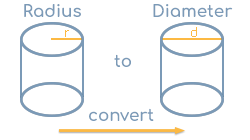Let a cylinder with a radius r of 2.5 in be given. We are looking for the diameter d of the cylinder.

#### Calculation

The diameter d corresponds to twice the radius r, i.e., d = 2 × r.

If the 2.5 in chosen in the example is used for the radius r, the diameter of the cylinder is d = 2 × 2.5 in = 5 in.

### How do you calculate the diameter of a cylinder from the circumference of the base?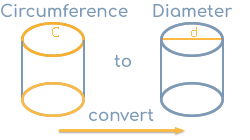Given is a cylinder with a circumference of the base C of 15 in. We are looking for the diameter d of the cylinder.

#### Calculation

The formula for the circumference of the base is C = d × π. If we convert this formula to d, the diameter d corresponds to the division of circumference of the base divided by pi (π = 3.1415...), thus d = C / π.

If the 15 in chosen in the example are used for the circumference of the base C, the diameter of the cylinder is d = 15 in / π ≈ 4.77 in.

### How do you calculate the diameter of a cylinder from the base area?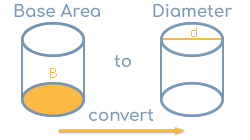Let a cylinder with a base area B of 20 in² be given. We are looking for the diameter d of the cylinder.

#### Calculation

The general formula for the base area B is B = r² × π. Since the diameter d corresponds to twice the radius r, the following formula applies: B = (d / 2)² × π. If we convert this formula to d, the diameter d is equal to twice the square root of the division of the base area B divided by Pi (π = 3.1415...), i.e., d = 2 × B / π.

Substituting the 20 in² chosen in the example for the base area B, the diameter of the cylinder is d = 2 × 20 in / π ≈ 5.05 in.

## 1.3 Calculate Cylinder Circumference of the Base

The following three examples illustrate how to calculate the circumference of the base of a cylinder using radius, diameter and base area.

### How do you calculate the circumference of the base of a cylinder from the radius?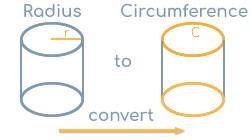Given a cylinder with a radius r of 2.5 in. We are looking for the cylinder circumference of the base C of the cylinder.

#### Calculation

The circumference of the base C corresponds to twice the radius r multiplied by the number Pi (π = 3.1415...), thus C = 2 × r × π.

If the 2.5 in chosen for the radius r in the example are used, the circumference of the base of the cylinder is C = 2 × 2.5 in × π ≈ 15.71 in.

### How do you calculate the circumference of the base of a cylinder from its diameter?Given a cylinder with a diameter d of 5 in. We are looking for the circumference of the base C of the cylinder.

#### Calculation

The circumference of the base C corresponds to the diameter d multiplied by the number Pi (π = 3.1415...), thus C = d × π.

Substituting the 5 in chosen in the example for the diameter d, the circumference of the base of the cylinder is C = 5 in × π ≈ 15.71 in.

### How do you calculate the circumference of the base of a cylinder from the base area?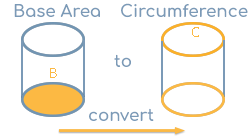Let a cylinder with a base area B of 20 in² be given. We are looking for the circumference of the base C of the cylinder.

#### Calculation

The general formula for the base area B is B = r² × π.

Using this formula, the radius r can now be calculated by converting the formula to r. The radius r then corresponds to the root of the result of the base area B divided by Pi (π = 3.1415...), i.e., r = G / π.

If we substitute the 20 in² chosen in the example for the base area B, the radius of the cylinder is r = 20 in / π ≈ 2.52 in.

#### Calculating the perimeter of the base

Using the radius calculated in this way, the general formula for calculating the circumference of the base can now be used: The circumference of the base C of the cylinder corresponds to twice the radius r multiplied by the number Pi (π = 3.1415...), i.e., C = 2 × r × π.

If we insert the previously calculated value for the radius r, the circumference of the base of the cylinder is C = 2 × 2.52 in × π ≈ 15.85 in.

## 1.4. Calculate Cylinder Base Area

The following three examples illustrate the calculation of the base area content using radius, diameter and circumference of the cylinder base.

### How do you calculate the base area of a cylinder from the radius?Let a cylinder with a radius r of 2.5 in be given. We are looking for the base area B of the cylinder.

#### Calculation

The base area B is equal to the square of the radius, i.e., r² multiplied by the number Pi (π = 3.1415...), and thus B = r² × π.

If we substitute the 2.5 in chosen in the example for the radius r, the base area of the cylinder is B = (2.5 in)² × π ≈ 19.63 in².

### How do you calculate the base area of a cylinder from its diameter?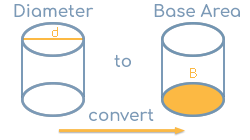Given a cylinder with a diameter d of 5 in. We are looking for the base area B of the cylinder.

#### Calculation

The base area B is equal to half the diameter squared, i.e., (d / 2)² multiplied by the number Pi (π = 3.1415...), and thus B = (d / 2)² × π.

If we insert the 5 in chosen in the example for the diameter d, the base area B of the cylinder is B = (5 in / 2)² × π ≈ 19.63 in².

### How do you calculate the base area of a cylinder from the circumference of the base?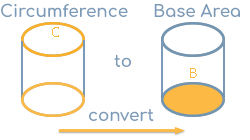Let a cylinder with a circumference of the base C of 15 in be given. We are looking for the base area B of the cylinder.

#### Calculation

The base area B is derived from the circumference, for example, by first determining the cylinder radius r based on the circumference of the base, because then you can use the common formula for the base area, namely B = r² × π.

So to calculate the radius first, you can rearrange the formula for the cylinder circumference of the base C = 2 × r × π to r, so that you get r = C / π / 2.

Substituting the 15 in chosen in the example for the circumference of the base C, the radius of the cylinder is r = 15 in / π / 2 ≈ 2.39 in.

#### Calculation of the base area

Finally, if we insert the value calculated here for the radius r, namely 2.39, into the general formula for the base area B = r² × π mentioned earlier, the base area of the cylinder is B = (2.39 in)² × π ≈ 17.90 in².

## 2.1. Calculate Cylinder Height

The following two examples illustrate the calculation of the cylinder height. The first example shows the calculation of the cylinder height using the base area and volume of the cylinder. The second example shows the calculation of the cylinder height using the base area and the surface area of the cylinder.

### How do you calculate the height of a cylinder from its base area and volume?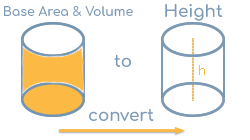Let a cylinder with a base area of 20 in² and a volume of 300 in³ be given. We are looking for the height h of the cylinder.

#### Calculation

The general formula for the volume V of a cylinder is V = B × h, where B is the base area and h is the height of the cylinder. If you convert this formula to h, you get h = V / B. The height h of a cylinder is therefore equal to the cylinder volume V divided by its base area B.

If the values chosen in the example for the volume V and the base area B are used, the height h is h = 300 in³ / 20 in² = 15 in.

### How do you calculate the height of a cylinder from its base area and lateral surface area?Given a cylinder with a base area of 20 in² and a lateral surface area of 200 in². We are looking for the height h of the cylinder.

#### Calculation

The rolled-up lateral surface (or curved surface) of a cylinder is a rectangle with the height of the cylinder and a width equal to the circumference of the cylinder. If you divide the given lateral surface area LSA by the cylinder circumference of the base C, you get its height. The formula for this is therefore h =  LSA / C.

#### Intermediate calculation of circumference of the base

The circumference of the base C is obtained from the given base area because, first, B = r² × π applies. Using this formula, the radius r can now be first calculated by converting the formula to r. The radius r then corresponds to the root of the result of the base area B divided by Pi (π = 3.1415...). The radius r then corresponds to the root of the result of the base area B divided by Pi (π = 3.1415...), i.e., r = B / π.

If the value entered for the cylinder base area B is used, the radius of the cylinder is r = 20 in² / π ≈ 2.52 in.

With the radius calculated in this way, the general calculation formula for the cylinder circumference of the base can now be used: The circumference of the base C corresponds to twice the radius r multiplied by the number Pi (π = 3.1415...), thus C =  2 × r × π.

If we insert the previously calculated value for the radius r, the circumference of the base area and thus of the cylinder is C = 2 × 2.52 in × π ≈ 15.85 in.

#### Calculation of the height

Finally, the value entered for the lateral surface area LSA and the calculated circumference of the base C are substituted into the formula for the height mentioned earlier. Using h =  LSA / C the cylinder height is therefore h = 200 in² / 15.85 in ≈ 12.62 in.

## 2.2. Calculate Cylinder Volume

The following two examples illustrate the calculation of the volume. The first example shows the calculation of the cylinder volume using the base area and height of the cylinder. The second example shows the calculation of the cylinder volume using the base area and the lateral surface area of the cylinder.

### How do you calculate the volume of a cylinder from its base area and height?Given a cylinder with a base area of 20 in² and a height of 15 in. We are looking for the volume V of the cylinder.

#### Calculation

The general formula for the volume V of a cylinder is V = B × h, where B is the base area and h is the height of the cylinder.

IIf we insert the values chosen in the example for the base area B and the height h, the volume V is therefore V = 20 in² × 15 in = 300 in³.

### How do you calculate the volume of a cylinder from its base area and lateral surface area?Given a cylinder with a base area of 20 in² and a lateral surface area of 250 in². We are looking for the height h of the cylinder.

#### Calculation

The general formula for the volume V of a cylinder is V = B × h, where B is the base area and h is the height of the cylinder. The base area B is given in the example. The height h of the cylinder can now be calculated using the given surface area:

The rolled-up lateral surface of a cylinder is a rectangle with the height of the cylinder and a width equal to the circumference of the cylinder base. If you divide the given lateral surface area LSA by the cylinder circumference of the base C, you get its height. The formula for this is therefore h =  LSA / B.

#### Intermediate calculation of the circumference

The circumference of the base C is obtained from the given base area, because B = r² × π applies first. With the help of this formula, the radius r can now first be calculated by converting the formula to r. The radius r then corresponds to the root of the result of the base area B divided by Pi (π = 3.1415...), i.e., r = B / π.

Substituting the entered value for the cylinder base area B, the radius of the cylinder is r = 20 in² / π ≈ 2.52 in.

With the radius calculated in this way, the general calculation formula for the cylinder circumference of the base can now be used: The circumference of the base C is equal to twice the radius r multiplied by the number Pi (π = 3.1415...), thus C = 2 × r × π.

If we insert the previously calculated value for the radius r, the circumference of the base area and thus of the cylinder is C = 2 × 2.52 in × π ≈ 15.85 in.

#### Intermediate calculation of height

Finally, using the value entered for the lateral surface area LSA and the circumference of the base calculated above, the cylinder height h is therefore h = 250 in² / 15.85 in ≈ 15.77 in.

#### Calculation of the volume

The volume V of a cylinder, as mentioned earlier, finally corresponds to the product of the base area B and the height h of the cylinder, i.e., V = B × h.

Finally, if the values for the entered base area B and the height h just calculated from the given surface area are inserted here, the volume is therefore V = 20 in² × 15.77 in ≈ 315.39 in³.

## 2.3. Calculate Cylinder Lateral Surface Area

The following two examples illustrate the calculation of the curved (lateral) surface area. The first example shows the calculation of the lateral surface area using the base area and height of the cylinder. The second example shows the calculation of the lateral surface area using the base area and the volume of the cylinder.

### How do you calculate the lateral surface area of a cylinder from its base area and height?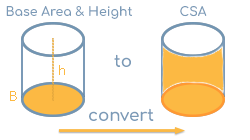Given a cylinder with a base area of 20 in² and a height of 15 in. We are looking for the lateral surface area (LSA) of the cylinder.

#### Calculation

The rolled-up lateral surface area LSA of a cylinder is a rectangle with the height h of the cylinder and a width equal to the circumference of the base C of the cylinder. Therefore, the formula LSA = h × C applies.

#### Intermediate calculation of circumference of the base

The circumference of the base C is obtained on the basis of the given base area, because the following first applies: B = r² × π. Using this formula, the radius r can now be first calculated by converting the formula to r. The radius r then corresponds to the root of the result of the base area B divided by Pi (π = 3.1415...), i.e., r = B / π.

If we insert the entered value for the cylinder base area B, the radius of the cylinder is r = 20 in² / π ≈ 2.52 in.

With the radius calculated in this way, the general formula for calculating the circumference of the cylinder base can now be used: The circumference of the base C corresponds to twice the radius r multiplied by the number Pi (π = 3.1415...), thus C =  2 × r × π.

Substituting the previously calculated value for the radius r, the circumference of the base area and thus of the cylinder is C = 2 × 2.52 in × π ≈ 15.85 in.

#### Calculation of lateral surface area

If the values entered for the height h and the circumference of the base C calculated in this way are substituted, the lateral surface area LSA is finally LSA = 15 in × 15.85 in ≈ 237.80 in².

### How do you calculate the lateral surface area of a cylinder from its base area and volume?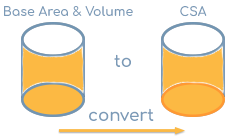Given a cylinder with a base area of 20 in² and a volume of 300 in³. We are looking for the lateral surface area LSA of the cylinder.

#### Calculation

The rolled-up lateral surface area LSA of a cylinder is a rectangle with the height h of the cylinder and a width equal to the circumference of the base C of the cylinder. The lateral surface area LSA can be calculated using the formula LSA = h × C.

#### Intermediate calculation of the circumference of the base

The circumference of the base C is obtained from the given base area because, first, B = r² × π applies. Using this formula, the radius r can now be calculated first by rearranging the formula according to r. The radius r then corresponds to the root of the result of the base area B divided by Pi (π = 3.1415...), i.e., r = B / π.

Substituting the entered value for the cylinder base area B, the radius of the cylinder is r = 20 in² / π ≈ 2.52 in.

With the radius calculated in this way, the general formula for calculating the circumference of the cylinder base can now be used: The circumference of the base C corresponds to twice the radius r multiplied by the number Pi (π = 3.1415...), thus C = 2 × r × π.

Substituting the previously calculated value for the radius r, the circumference of the base area and thus of the cylinder is C = 2 × 2.52 in × π ≈ 15.85 in.

#### Intermediate calculation of height

The height h can be calculated for the given volume V and the given base area B using the following formula: h = V / B.

If we insert the given volume V and the base area B, the height is thus h = 300 in³ / 20 in² = 15 in.

#### Calculation of the lateral surface area

With the height h calculated in this way and the circumference of the base C of the cylinder already determined, the lateral surface area LSA can now be calculated using the formula LSA = h × C be calculated.

If we insert the calculated values for the height h and the circumference of the base C, the lateral surface area is LSA = 15 in × 15.85 in ≈ 237.80 in².

## 2.4. Calculate Cylinder Total Surface Area

The following final example shows the calculation of the total surface area of a cylinder based on its base area and lateral surface area.

### How do you calculate the total surface area of a cylinder from its base area and lateral surface area?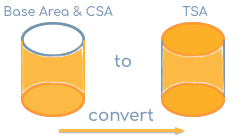Given a cylinder with a base area of 20 in² and a lateral surface area of 200 in². We are looking for the total surface area (TSA) of the cylinder.

#### Calculation

The total surface area TSA is made up of twice the base area B and the lateral surface area LSA, i.e., LSA = 2 × B + CSA.

If we use the 20 in² chosen in the example for the base area B and 200 in² for the lateral surface area LSA, the total surface area TSA of the cylinder is therefore TSA = 2 × 20 in² + 200 in² = 240 in².

## Source information

As source for the information in the 'Cylinder' category, we have used in particular:

## Last update on March 14, 2023

The page of the 'Cylinder' category was last changed by Michael Mühl on March 14, 2023. In addition, the page is continuously reviewed editorially and corresponds to the current status.

### Changes in this category "Cylinder"

• 14.03.2023: Publication of the Cylinder Calculation together with the corresponding texts.